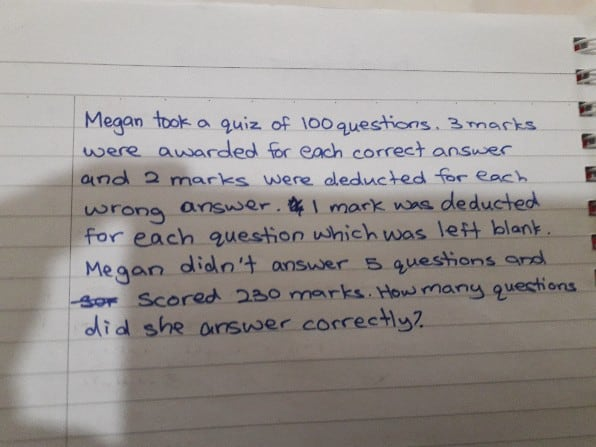# QuestionHow to do this question

Thank u

Marks gained for correct answer = 3

Marks lost for incorrect answer = 5

Marks lost for blanks = 4

Solve by assumption method

Let us assume that all responses are correct.

In that case, Total marks would be = 100 x 3 = 300.

Marks lost due to blanks = 5 x 4  = 20

Marks after deducting for blanks = 300 – 20  = 280.

She scored only 230. So marks she lost = 280 – 230 = 50.

50 marks were lost due to incorrect answers.

So, number of incorrect answers = 50 /5 = 10.

Number of correct answers = 100 – 10 – 5 = 85

Verify